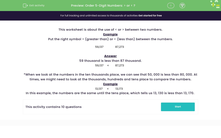# Order 5-Digit Numbers: > or < ?

In this worksheet, students use the symbols > and < correctly between two large numbers.Key stage:  KS 2

Curriculum topic:   Number: Number and Place Value

Curriculum subtopic:   Know Numbers to 1 000 000

Popular topics:   Numbers worksheets

Difficulty level:#### Worksheet Overview

This worksheet is about the use of < or > between two numbers.

Example

Put the right symbol > (greater than) or < (less than) between the numbers.

59,137    87,273

59 thousand is less than 87 thousand.

59,137
 <
87,273

*When we look at the numbers in the ten thousands place, we can see that 50, 000 is less than 80, 000. At times, we might need to look at the thousands, hundreds and tens place to compare the numbers.

Example

13,137
 <
13,173

In this example, the numbers are the same until the tens place, which tells us 13, 130 is less than 13, 170.

### What is EdPlace?

We're your National Curriculum aligned online education content provider helping each child succeed in English, maths and science from year 1 to GCSE. With an EdPlace account you’ll be able to track and measure progress, helping each child achieve their best. We build confidence and attainment by personalising each child’s learning at a level that suits them.

Get started